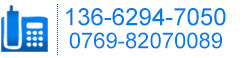news-3

（1200+600+800）单边工作绐（1200+600+800）单边工作台
（1200+750+800）单边工作台
（1500+750+800）单边工作台
（1200+750+800）单边工作台
（1500+750+800）单边工作台
（1800+750+800）双边工作台
（1200+600+800）双边工作台
（1200+750+800）双边工作台
（1500+750+800）双边工作台
（1200+750+800）双边工作台
（1500+750+800）双边工作台
（1800+750+800）双边工作台
（1800+750+800）双边工作绐

-THE END-